# Types of Chemical Reactions 5 basic types of

• Slides: 24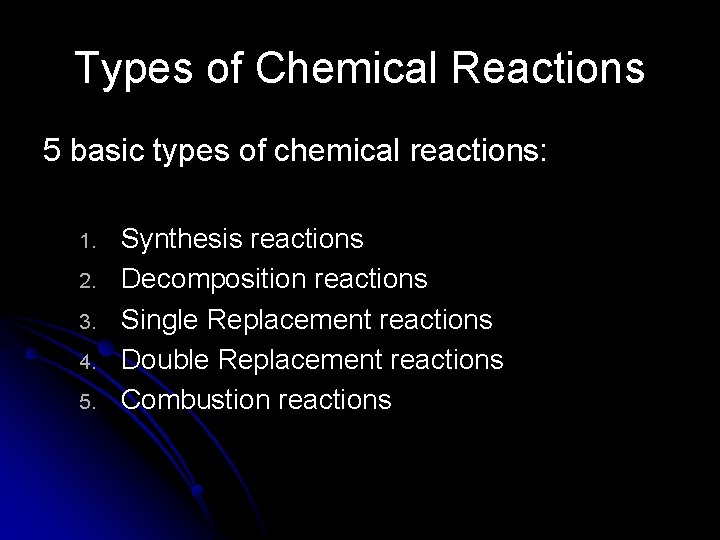Types of Chemical Reactions 5 basic types of chemical reactions: 1. 2. 3. 4. 5. Synthesis reactions Decomposition reactions Single Replacement reactions Double Replacement reactions Combustion reactionsTypes of Reactions • You need to be able to identify the type of reaction and balance reaction • Honors Students need to also predict product(s)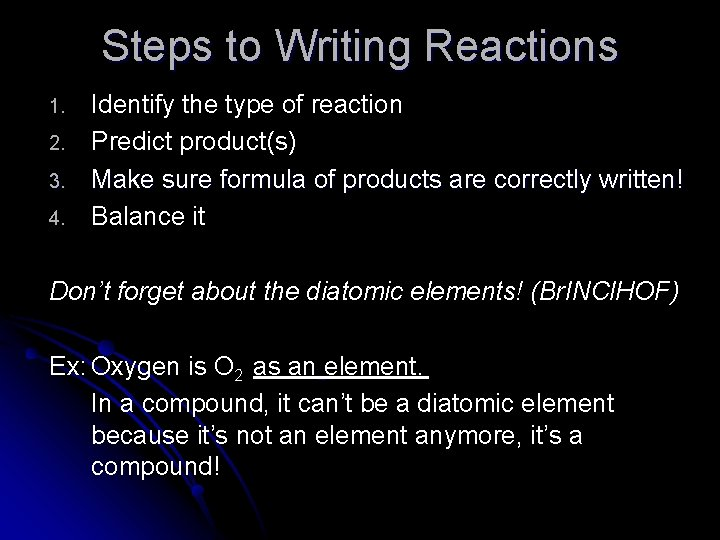Steps to Writing Reactions 1. 2. 3. 4. Identify the type of reaction Predict product(s) Make sure formula of products are correctly written! Balance it Don’t forget about the diatomic elements! (Br. INCl. HOF) Ex: Oxygen is O 2 as an element. In a compound, it can’t be a diatomic element because it’s not an element anymore, it’s a compound!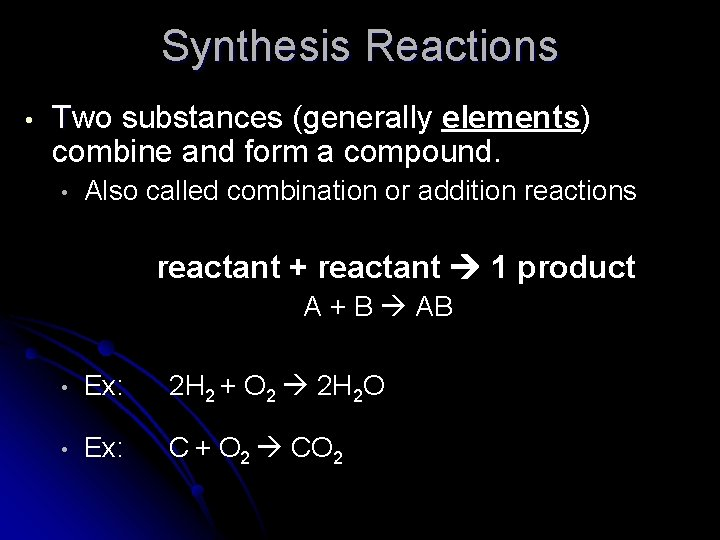Synthesis Reactions • Two substances (generally elements) combine and form a compound. • Also called combination or addition reactions reactant + reactant 1 product A + B AB • Ex: 2 H 2 + O 2 2 H 2 O • Ex: C + O 2 CO 2Synthesis Reactions • Another example of a synthesis reaction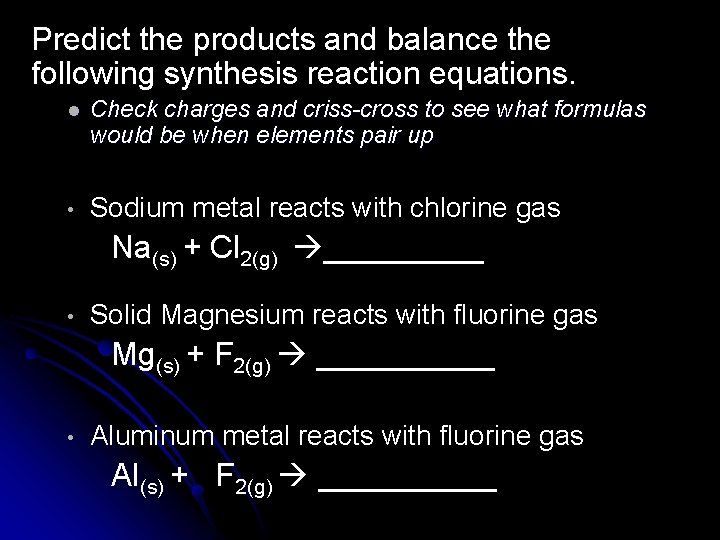Predict the products and balance the following synthesis reaction equations. l Check charges and criss-cross to see what formulas would be when elements pair up • Sodium metal reacts with chlorine gas Na(s) + Cl 2(g) _____ • Solid Magnesium reacts with fluorine gas Mg(s) + F 2(g) _____ • Aluminum metal reacts with fluorine gas Al(s) + F 2(g) _____Decomposition Reactions A compound breaks up into elements or into a few simpler compounds 1 Reactant Product + Product AB A + B • Ex: 2 H 2 O 2 H 2 + O 2 • Ex: 2 Hg. O 2 Hg + O 2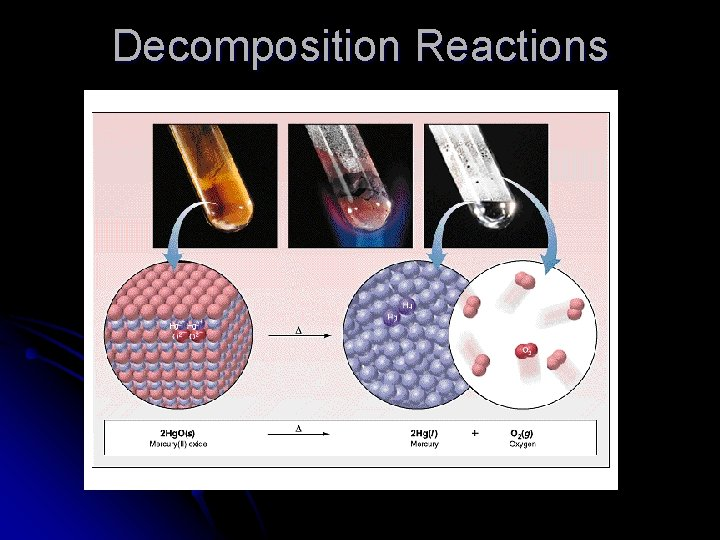Decomposition ReactionsDecomposition Exceptions (Honors) • Carbonates and chlorates are special case decomposition reactions that do not break down to elements • Carbonates (CO 32 -) decompose to carbon dioxide and a metal oxide • • Example: Ca. CO 3 CO 2 + Ca. O Chlorates (Cl. O 3 -) decompose to oxygen gas and a metal chloride • Example: 2 Al(Cl. O 3)3 2 Al. Cl 3 + 9 O 2Practice • • Predict the products Write formulas of products correctly (Remember diatomic elements!!) Balance the following decomposition reactions • Pb. O 2(s) ___________ • Al. N(s) ____________Single Replacement Reactions • One element replaces another in a compound. • A metal can replace a metal (+) OR a nonmetal can replace a nonmetal (-) element + compound A + BC AC + B (if A is a metal) OR A + BC BA + C (if A is a nonmetal) (remember the cation always goes first!)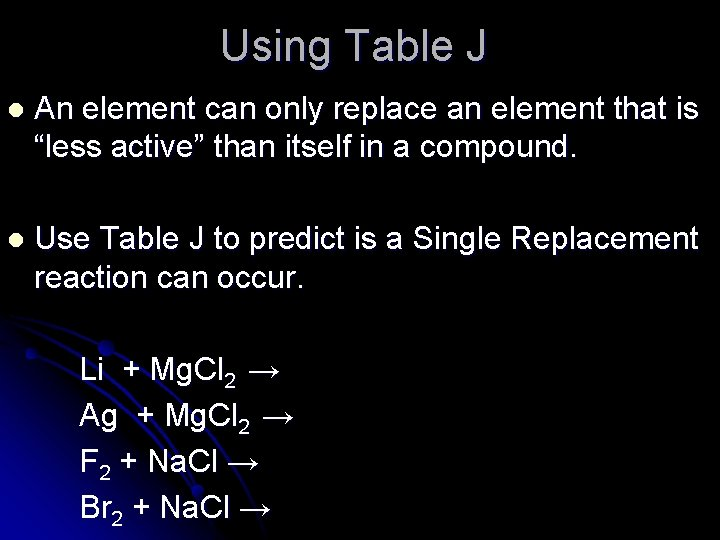Using Table J l An element can only replace an element that is “less active” than itself in a compound. l Use Table J to predict is a Single Replacement reaction can occur. Li + Mg. Cl 2 → Ag + Mg. Cl 2 → F 2 + Na. Cl → Br 2 + Na. Cl →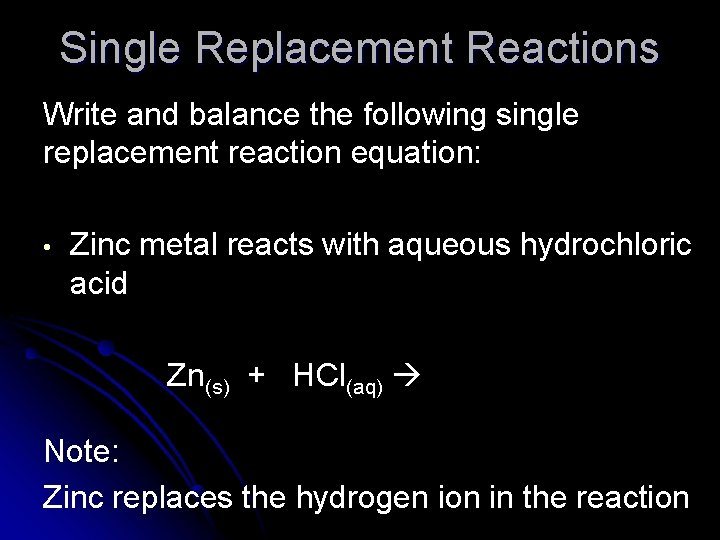Single Replacement Reactions Write and balance the following single replacement reaction equation: • Zinc metal reacts with aqueous hydrochloric acid Zn(s) + HCl(aq) Note: Zinc replaces the hydrogen ion in the reactionSingle Replacement Reactions • Sodium chloride solid reacts with fluorine gas Na. Cl(s) + F 2(g) _______ • Aluminum metal reacts with copper (II) nitrate(aq) Al(s)+ Cu(NO 3)2(aq) ________Double Replacement Reactions A metal replaces a metal in a compound a nonmetal replaces a nonmetal in a compound • • Ions “switch partners” Forms and insoluble precipitate and/or water Compound + compound+ compound AB + CD AD + CBDouble Replacement Reactions • First & last ions go together, inside ions go together. • All ions keep their original charges with new partners • Ex: Ag. NO 3(aq) + Na. Cl(s) Ag. Cl(s) + Na. NO 3(aq) • Ex: K 2 SO 4(aq) + Ba(NO 3)2(aq) 2 KNO 3(aq) + Ba. SO 4(s)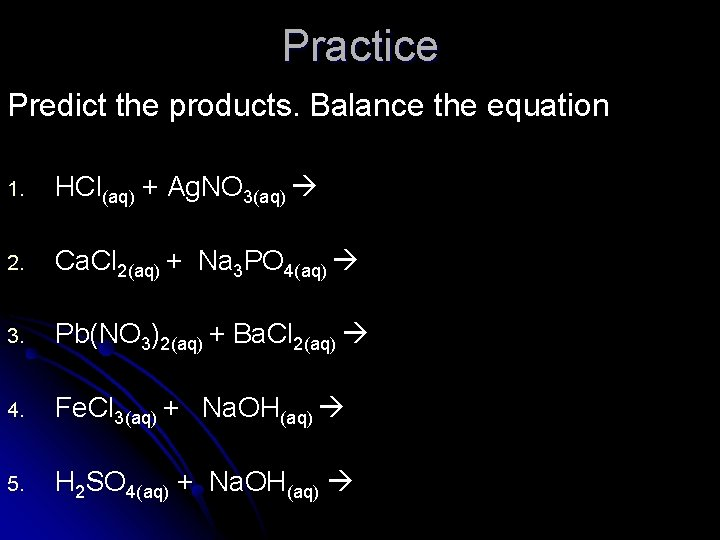Practice Predict the products. Balance the equation 1. HCl(aq) + Ag. NO 3(aq) 2. Ca. Cl 2(aq) + Na 3 PO 4(aq) 3. Pb(NO 3)2(aq) + Ba. Cl 2(aq) 4. Fe. Cl 3(aq) + Na. OH(aq) 5. H 2 SO 4(aq) + Na. OH(aq)Combustion Reactions • A hydrocarbon reacts with oxygen gas. • This is also called burning!!! • In order to burn something you need the 3 things in the “fire triangle”: l l l Fuel (hydrocarbon) Oxygen to burn it with Something to ignite the reaction (spark)Combustion Reactions • In general: Cx. Hy + O 2 CO 2 + H 2 O • Products in combustion are ALWAYS carbon dioxide and water. • • Although incomplete burning does cause some by-products like carbon monoxide (CO) and soot (solid carbon) Combustion is used to heat homes and run automobiles • Ex: Octane, as in gasoline, is C 8 H 18Combustion • C 5 H 12 + O 2 CO 2 + H 2 O • Write products and balance the following combustion reaction: C 10 H 22 + O 2Mixed Practice State the type, predict the products, and balance the following reactions: TYPE 1. _____ Ba. Cl 2 + H 2 SO 4 2. _____ C 6 H 12 + O 2 3. _____ Zn + Cu. SO 4 4. _____ Cs + Br 2 5. _____ Fe. Cl 3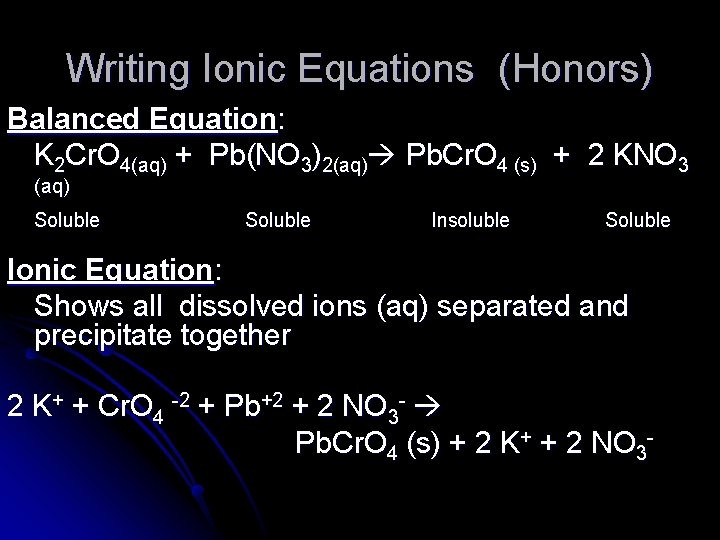Writing Ionic Equations (Honors) Balanced Equation: K 2 Cr. O 4(aq) + Pb(NO 3)2(aq) Pb. Cr. O 4 (s) + 2 KNO 3 (aq) Soluble Insoluble Soluble Ionic Equation: Shows all dissolved ions (aq) separated and precipitate together 2 K+ + Cr. O 4 -2 + Pb+2 + 2 NO 3 - Pb. Cr. O 4 (s) + 2 K+ + 2 NO 3 -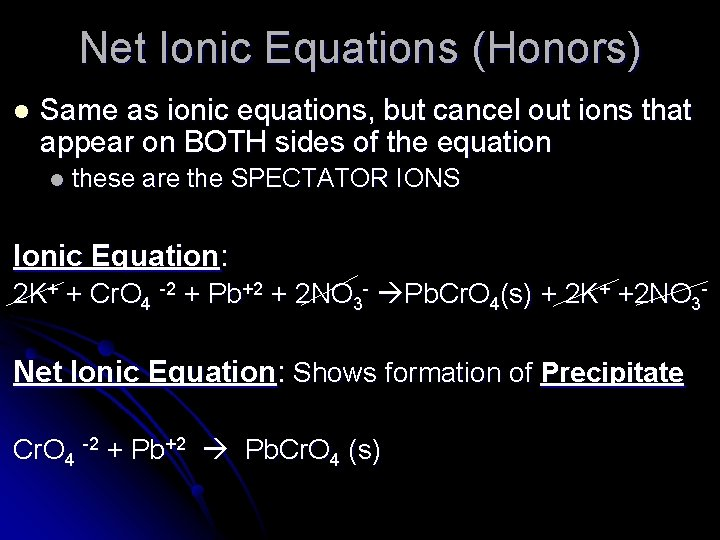Net Ionic Equations (Honors) l Same as ionic equations, but cancel out ions that appear on BOTH sides of the equation l these are the SPECTATOR IONS Ionic Equation: 2 K+ + Cr. O 4 -2 + Pb+2 + 2 NO 3 - Pb. Cr. O 4(s) + 2 K+ +2 NO 3 - Net Ionic Equation: Shows formation of Precipitate Cr. O 4 -2 + Pb+2 Pb. Cr. O 4 (s)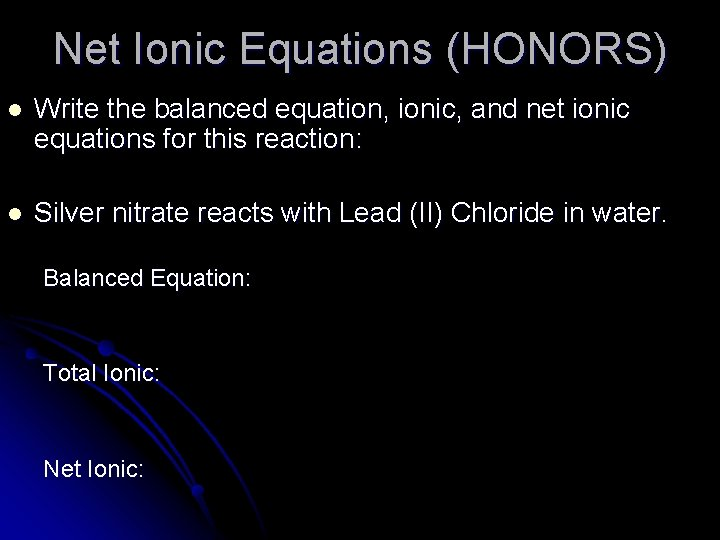Net Ionic Equations (HONORS) l Write the balanced equation, ionic, and net ionic equations for this reaction: l Silver nitrate reacts with Lead (II) Chloride in water. Balanced Equation: Total Ionic: Net Ionic: